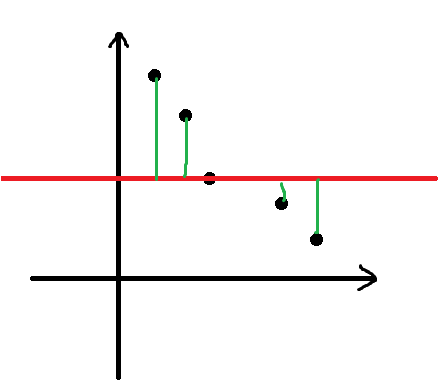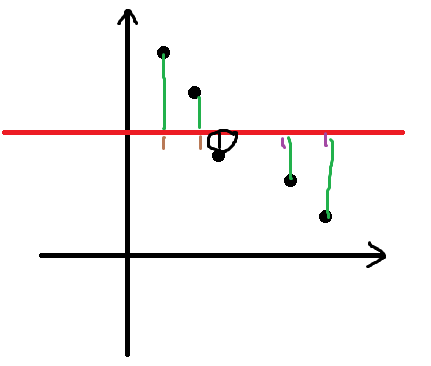# BZOJ[Baltic2004]Sequence 可并堆

①若$t_1<t_2<t_3<…<t_n$

②若$t_1>t_2>t_3>…>t_n$#include<algorithm>
#include<cstdlib>
#include<cstdio>
#define N 1000600
using namespace std;
int size[N],ch[N],a[N],root[N],l[N],r[N],tot[N],top,n;
long long ans=0ll;
int merge(int x,int y){
if(!x) return y;if(!y) return x;
if(a[x]<a[y]) swap(x,y);
ch[x]=merge(ch[x],y);
size[x]=size[ch[x]]+size[ch[x]]+1;
swap(ch[x],ch[x]);
return x;
}
int main(){
scanf("%d",&n);
for(int i=1;i<=n;i++) scanf("%d",a+i),a[i]-=i;//要求单调递增
for(int i=1;i<=n;i++){//root:这段区间的中位数
l[++top]=r[top]=root[top]=i;//l:这段区间包含的最左边的点,r：这段区间包含的最右边的点
tot[top]=size[i]=1;//tot:这个区间包含多少个数 size:
while(top>1&&a[root[top-1]]>a[root[top]]){
r[--top]=r[top+1]; tot[top]+=tot[top+1];
root[top]=merge(root[top],root[top+1]);//将这个点合并到上一个序列里
//下面的过程是将中位数调到堆顶(不断删除堆顶)
while(size[root[top]]*2>tot[top]+1) root[top]=merge(ch[root[top]],ch[root[top]]);
}
}
for(int i=1;i<=top;i++)
for(int j=l[i];j<=r[i];j++)
ans+=(long long)abs(a[j]-a[root[i]]);
return printf("%lld",ans),0;
}


posted @ 2018-03-30 09:19  Duan2baka  阅读(110)  评论(0编辑  收藏  举报